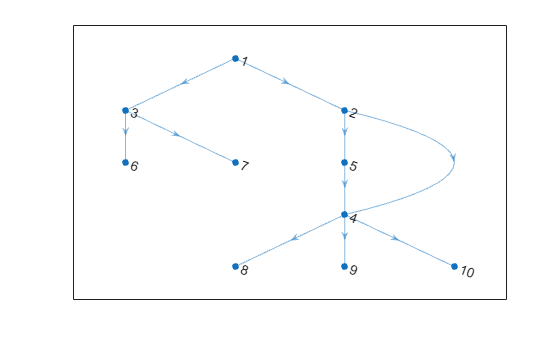# isdag

Determine if graph is acyclic

## Syntax

``tf = isdag(G)``

## Description

example

````tf = isdag(G)` returns logical `1` (`true`) if `G` is a directed acyclic graph; otherwise, it returns logical `0` (`false`).```

## Examples

collapse all

Create and plot a directed graph, and then test the graph to determine if it is acyclic.

```s = [1 1 2 2 3 3 4 4 4 5]; t = [2 3 4 5 6 7 8 9 10 4]; G = digraph(s,t)```
```G = digraph with properties: Edges: [10x1 table] Nodes: [10x0 table] ```
`plot(G)``tf = isdag(G)`
```tf = logical 1 ```

## Input Arguments

collapse all

Input graph, specified as a `digraph` object. Use `digraph` to create a directed graph object.

Example: `G = digraph([1 2],[2 3])`